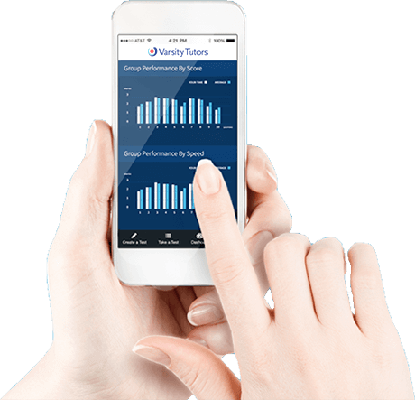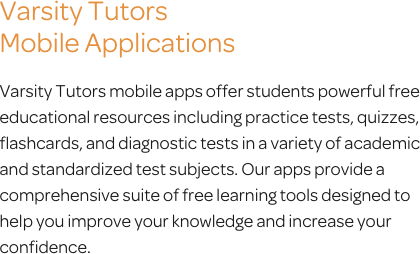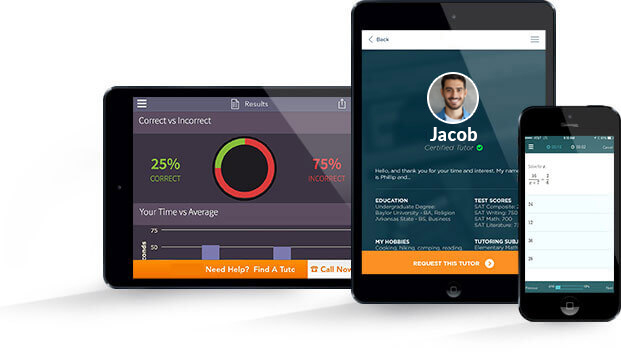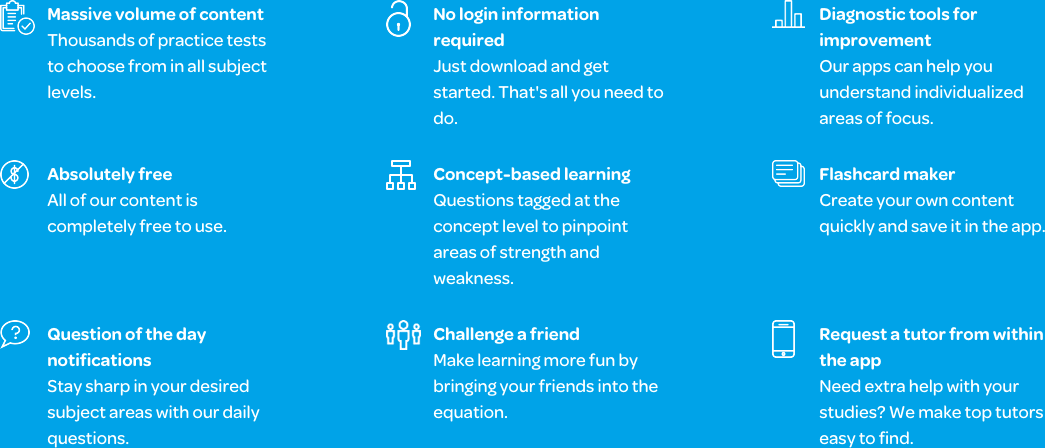The Varsity Tutors Algebra 2 Mobile App

Algebra 2 is the course students generally take following Algebra 1. It moves on from algebraic basics to concepts that students need to know in order to be able to learn trigonometry or calculus. Parabolic functions, hyperbolic functions, and quadratic inequalities will be covered later, but the concepts learned now are essential for future success in these areas. Students will soon need to factor polynomials and use logarithms and exponents. Guidance and support can be found with the free Varsity Tutors Algebra 2 app for Android, iPhone, and iPad, available from iTunes and the Google Play Store.

Students typically encounter Algebra 2 in the first half of high school. Prior to taking this class, they are expected to have mastered working with single-variable equations, graphs, and functions. The more advanced concepts taught in Algebra 2 prepare students to work with trigonometry, pre-calculus, and calculus. For now, their practice needs to focus on understanding how to represent data. A familiarity with the relationships between various aspects of data are important, so Algebra 2 usually begins with a review of topics students have already learned.

Students at this level move beyond the simple functions they have already learned. Rather than single-variable equations, they work with quadratic equations, advanced graphs, and polynomials. The hundreds of problems, flashcards, and practice tests available through the app have been created by experts in the field, and provide many chances for reviewing the subject matter.

Students can practice concepts such as working with irrational numbers, factoring radicals, working with the quadratic formula, and assessing probability. They can download and review flashcards, and even create their own flashcards with custom text, images, and audio. These can help them better understand equations and graphical functions. The flashcards also enable students to practice working with numerical and graphical relationships. This extra review gives students the ability to understand how changes in graphical values are reflected in equations. The flashcards also provide practice using variations of equations, interpreting these changes on a graph, and how to arrive at the correct answer the first time.

The concept-specific and full-length practice tests on the app let students concentrate on material that will be covered on in-school quizzes and tests. Quick practice is possible with the iPhone, iPad, and Android app, but one can also focus on independent learning. All of the questions’ answers are explained in detail. If a student misses the right answer, the thorough explanations will show them how to find the correct response. Students can also track their progress, share their scores, and learn along the way.

Whether they have yet to learn a topic, or just need to brush up on the concepts, the practice tests, flashcards, and other tools available through the app can help. The questions are written by professional educators and offer a direct approach to assessing students’ abilities. Armed with that knowledge, students can concentrate on strengthening the skills they may not have mastered yet. As more and more complex mathematical material is covered in later classes, it’s important that students take the time to practice Algebra 2 concepts now. The free Varsity Tutors Algebra 2 app for Android, iPhone, and iPad can be downloaded from Google Play or iTunes, providing students with supplemental materials, expertly created resources, and valuable guidance.Algebra II is a step above the basic content you learned in Algebra I. You will transition from working with simple numbers and symbols, and the mechanics of basic equations, to more technical content that will enable you to cross the bridge over to the more advanced subjects of pre-calculus and calculus. Although algebra II is just another step up, it paves the way to higher mathematics and possibly careers in science and engineering.

The most essential thing to know about learning algebra II is that mastering it helps prepare one for the rigors of college. It is a tool for reasoning. You might find yourself using the symbols and equations, or at least the mental skills, to manage finances or plan a road trip. The subject is the very foundation of calculus, so each lesson is a stepping stone to greater things to come. You’ll learn problem-solving skills that expand your abilities and also build self-confidence. First, however, some basic fundamentals (they may not seem so at first) such as the arithmetic sequence must be conquered. These involve continuously adding values to the previous terms. Beyond the most basic form, you’ll be working with values in parentheses, fractions, and eventually whole equations.

All through school, you’ve been taught to think rationally. In algebra II you’ll have to come to terms with irrational numbers. These cannot be created by dividing two integers; the infamous Pi, or 3.14… is a perfect example. You’ll move on to do intricate arithmetic operations with these numbers and expressions. Fractions will of course play into all of this.

Algebra II covers linear and quadratic equations, roots, radicals, and exponential functions. As you advance through the course, you’ll encounter logarithmic functions, conic sections, probability, and trigonometry. Functions are one of the most important concepts to consider. You’ll need to understand the relations between variables in order to calculate the output value from the input value. As simple as they seem at this level, functions appear throughout higher mathematics, so it’s important to master them now. Finding the inverse of a function is another procedure, and comes in the form of an equation. You will learn how to algebraically derive the inverse of a quadratic function and even graph the formula. With this, you can make connections and find the average rate of change, for example.

Other algebra II topics include graphing. You might be used to solving equations and working with basic algebra. A more visual approach to this is to plot equations and functions on a graph. You’ll have to identify the x and y axis and where positive and negative values are appropriate. Polynomials come into play and enable you to perform more complex operations, with quadratic equations, for example. Expanding on the principles of square roots you learned in prior classes are roots and radicals. Algebra II brings in cube roots, fifth roots, and beyond, and you’ll work with many symbols and operations with roots, enabling you to perform even higher-order operations.

With conic sections, you’ll be able to create slices through cones at various angles and make calculations. This has many real-world applications. In astronomy, it’s used to calculate planetary orbits; in engineering, it’s used in the design and shaping of satellite dishes. Simple mathematical operations are limited in how you can add, subtract, divide, and multiply. Exponents expand on what you can do with numbers. In algebra II, you’ll be exposed to working with exponential functions. Logarithms provide mathematical tools to work in the inverse. The more you work with these concepts, the easier and faster you’ll be able to analyze and solve problems and equations.

The field of systems and inequalities comes into play in algebra II. As you move forward, you should be able to use just one variable to solve an inequality and be able to work with linear and non-linear graphing systems. Quadratics enable you to do higher order operations with squares and cubes. You’ll be introduced to concepts such as imaginary numbers. Solving quadratic problems will yield complex answers, and you’ll need to learn how to recognize and arrive at these, recognizing when they are legitimate solutions. The ability to work with negative numbers is paramount, since the square root functions you learned earlier will yield negative results. Such numbers don’t seem possible, but have many applications in solving real problems.

Statistics is another component of algebra II. It involves working with mean and standard deviation, normal distribution, and areas under normal distribution. Random sampling and margin of error will be added to your list of acquired capabilities. It’ll also be up to you to define statistical values and practical elements and, of course, you’ll have to explain how and why you arrived at a specific conclusion.

The world of trigonometry will be at your fingertips as you advance through algebra II. It involves working with the right triangle, the hypotenuse, and opposite and adjacent sides. Eventually, any type of angle can be factored in, and you’ll need to choose and work with the appropriate trig functions. Once you grasp the patterns and have everything memorized, it’ll be quicker to make calculations. Specific formulas include reciprocal identities, tangent and cotangent identities, and sum and difference formulas. Domain, range, and period are essential concepts. Each individual symbol and formula has significant meaning and is essential to know in order to master trigonometry. Once you get past radian measure, arc length, and are able to graph trig functions with amplitude, mid-line, and other applications, it should become much clearer as to where it is all going mathematically.

In algebra II, you’ll also encounter cool things such as the Fibonacci sequence, Pascal’s triangle, subsets, and partial sums. Logic and matrices will give you even more tools to harness your brain power to advance in the world of mathematics.

Algebra II involves a lot of memorization. You also must learn to work with each formula and know how to apply them in the appropriate applications. The skills you will learn take a lot of practice and perseverance, but your hard work will prepare you for more advanced math concepts down the road.